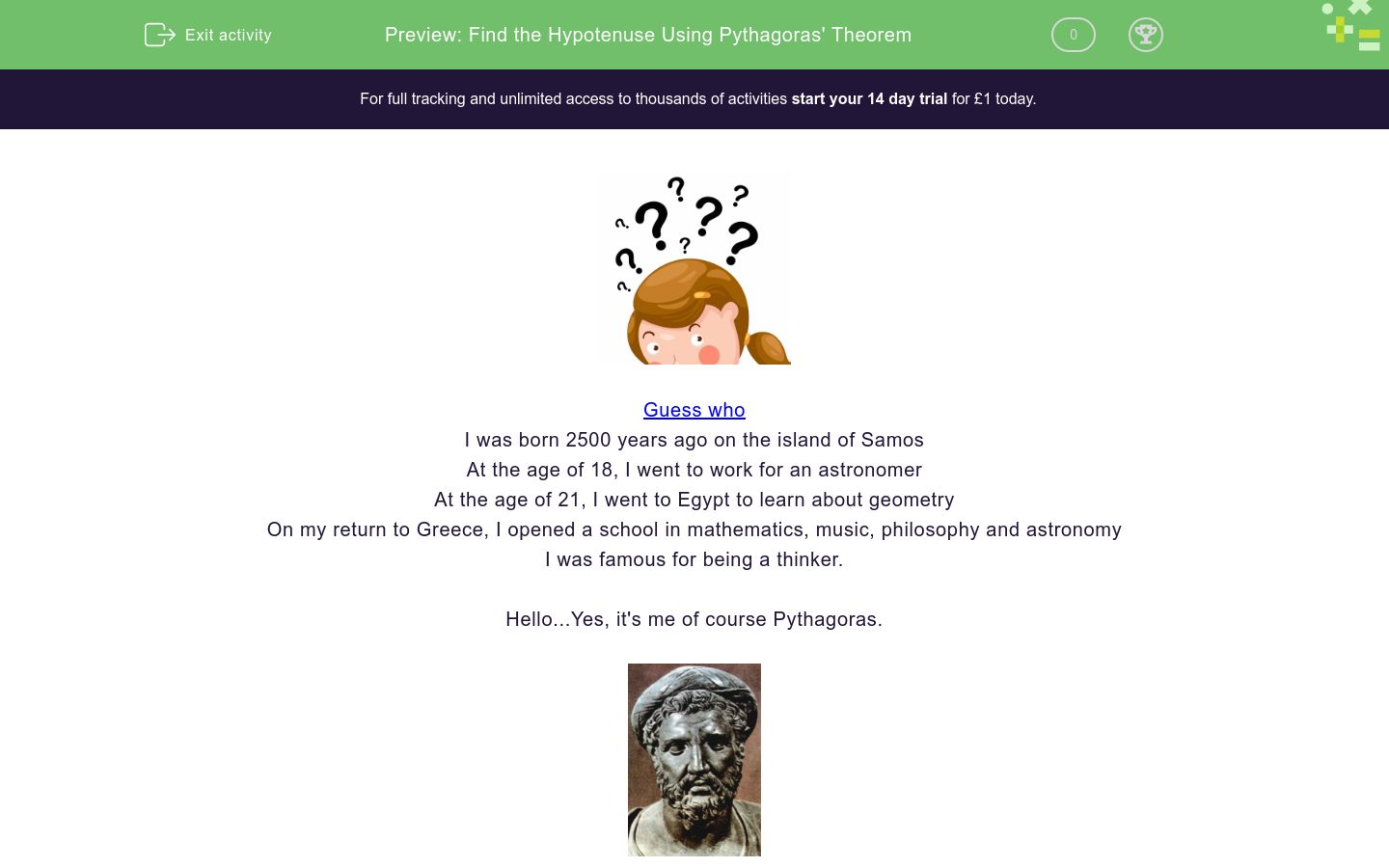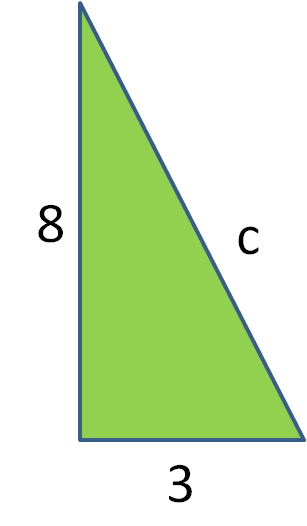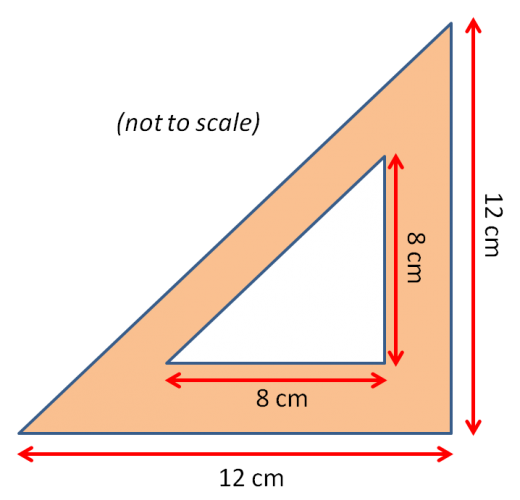# Find the Hypotenuse Using Pythagoras' Theorem

In this worksheet, students will find the length of a hypotenuse of a triangle using Pythagoras' Theorem.Key stage:  KS 4

GCSE Subjects:   Maths

GCSE Boards:   AQA, Eduqas, Pearson Edexcel, OCR

Curriculum topic:   Geometry and Measures, Mensuration

Curriculum subtopic:   Mensuration and Calculation, Triangle Mensuration

Difficulty level:### QUESTION 1 of 10Guess who

I was born 2500 years ago on the island of Samos

At the age of 18 I went to work for an astronomer

At the age of 21 I went to Egypt to learn about geometry

On my return to Greece I opened a school in mathematics, music, philosophy and astronomy

I was famous for being a thinker.

Hello.....Yes its me of course Pythagoras.I am going to introduce you to my most well known theorem.

Pythagoras' Theorem is a way of finding the length of the hypotenuse of a right angled triangle.Find the length of c

I bet your teacher has told you the proper formula which can get very confusing.

Forget the formula,  just follow these steps.

1.  Take the number from 1 side and square it.  2 x 2 = 4

2.  Take the number from the other side and square it. 6 x 6 = 36

4. Square root (√) 40 = 6.32 to 2 decimal places.By the way, this only works for right angled triangles.Check

The hypotenuse is always the longest side of a triangle.

So if you answer is smaller than any of the other sides you have made a mistake.

The formula if you want to know is a² + b² = c²What do you call a pot of boiling water at the top of Mount Everest?

High Pot in Use.

What is the length of C?

The answer has been rounded to 2 decimal places.7.54

5.84

6.54

8.54Calculate the length of C.What is the value of c?

Match up

## Column B

Right angled triangle with sides 4 cm and 5 cm
Hypotenuse is 9.22 cm
Right angled triangle with sides 3 cm and 5 cm
Hypotenuse is 6.71
Right angled triangle with sides 6 cm and 7 cm
Hypotenuse is 5.83 cm
Right angled triangle with sides 6 cm and 3 cm
Hypotenuse is 6.40 cmWhat is the length of the diagonal of this triangle?

The length of the rectangle is 8 cm and the width is 4 cm.

 8.58 cm² 8.38 cm² 9.28 cm² 8.94 cm² Length of diagonalThe base of this triangle is 12 cm and the height is 8 cm.

What is the length of the slanted side?

Did you know that the size of a TV screen is determined using Pythagoras Theorem?

The size is  measured by the the hypotenuse of the television.What is the size of this TV Screen?

The base is 45 inches  and the height is 30 inches

34 inches

44 inches

54 inches

24 inchesWhat is the screen size of this smart phone?

Base is 4 cm  and the height is 7 cm.

34 inches

44 inches

54 inches

24 inches12.31 cm 16.97 cm 11.31 cm 17.25 cm Hypotenuse of white triangle Hypotenuse of orange triangle.
• Question 1

What is the length of C?

The answer has been rounded to 2 decimal places.8.54
EDDIE SAYS
So did you find not trying to use the formula easier. 8² + 3² = 73 √73 = 8.54 Pythagoras was a top bloke you know.
• Question 2Calculate the length of C.

7.07
EDDIE SAYS
6 x 6 = 36 4 x 4 = 16 36 + 16 = 50 √50 = 7.07 Did you know Pythagoras never wrote down his famous theory? He must have been clever.
• Question 3EDDIE SAYS
Square, square, add and square root. Thats all there is to it. SSAS 7 x 7 = 49 5 x 5 = 24 49 + 25 = 74 √74 = 8.60
• Question 4What is the value of c?

10.44
EDDIE SAYS
Square, Square, Add, Square root You can write you answers in various ways such as 3 x 3 = 9 10 x 10 = 100 100 + 109 = 109 √ 109 = 1.44 Or 3² + 10² = √109 = 10.44 Pythagoras wouldn't mind either way.
• Question 5

Match up

## Column B

Right angled triangle with sides ...
Hypotenuse is 6.40 cm
Right angled triangle with sides ...
Hypotenuse is 5.83 cm
Right angled triangle with sides ...
Hypotenuse is 9.22 cm
Right angled triangle with sides ...
Hypotenuse is 6.71
EDDIE SAYS
Are you checking that the hypotenuse is always longer than the other sides. This is a good way to check if you are correct Square side 1 + square side 2. Add your answers together. √ your answer
• Question 6What is the length of the diagonal of this triangle?

The length of the rectangle is 8 cm and the width is 4 cm.

 8.58 cm² 8.38 cm² 9.28 cm² 8.94 cm² Length of diagonal
EDDIE SAYS
But this isn't a triangle I hear you say. Well spotted, all you have to do is draw the diagonal line in and you will spot the right angled triangle. 8² + 4² = √80² = 8.94 cm
• Question 7The base of this triangle is 12 cm and the height is 8 cm.

What is the length of the slanted side?

10
EDDIE SAYS
Hang on, this is not a right angled triangle. No problem, we can make it one. We just draw a line down from the point to the base to create two right angles triangles. We know the height is 8 cm BE careful here as we have been given the base of the triangle as 12 cm but as we have cut it in 2 we only want half which is 6 cm. 6² + 8² = 100 √100 = 10
• Question 8

Did you know that the size of a TV screen is determined using Pythagoras Theorem?

The size is  measured by the the hypotenuse of the television.What is the size of this TV Screen?

The base is 45 inches  and the height is 30 inches

54 inches
EDDIE SAYS
Imagine a diagonal line through the TV screen to create a right angled triangle. 30 x 30 = 900 inches 45 x 45 = 2025 inches 900 + 2025 = 2925 √5625 = 54 inches Wow thats a big TV. I wonder if all those years ago Pythagoras knew what his theorem would be used for.
• Question 9What is the screen size of this smart phone?

Base is 4 cm  and the height is 7 cm.

EDDIE SAYS
Just look on how to turn this into a right angled triangle. Yes drawing an imaginary line on the diagonal. You don't want to draw a real one as it would annoy you trying to read the screen. 7² + 4² = 65² √65 =8.06 cm
• Question 1012.31 cm 16.97 cm 11.31 cm 17.25 cm Hypotenuse of white triangle Hypotenuse of orange triangle.
EDDIE SAYS
See you can do two things at once now. Good old Pythagoras. He certainly knew what he was doing. 8² + 8² = 128 √ 128 = 11.31 cm 12² +12² = 288 √ 128 = 16.97 cm
---- OR ----

Sign up for a £1 trial so you can track and measure your child's progress on this activity.

### What is EdPlace?

We're your National Curriculum aligned online education content provider helping each child succeed in English, maths and science from year 1 to GCSE. With an EdPlace account you’ll be able to track and measure progress, helping each child achieve their best. We build confidence and attainment by personalising each child’s learning at a level that suits them.

Get started